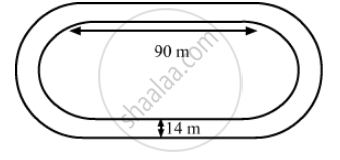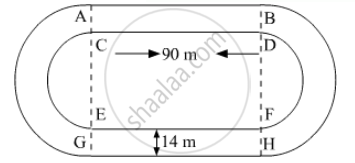Advertisement Remove all ads

# The Inside Perimeter of a Running Track (Shown in the Following Figure) is 400 M. the Length of Each of the Straight Portion is 90 M and the Ends Are Semi-circles. If the Track is Everywhe - Mathematics

Sum

The inside perimeter of a running track (shown in the following figure) is 400 m. The length of each of the straight portion is 90 m and the ends are semi-circles. If the track is everywhere 14 m wide. find the area of the track. Also find the length of the outer running track.Advertisement Remove all ads

#### Solution

It is given that, "length of each straight portion"and "width of track=14m"We know that the circumference C of semicircle of radius be r is $C = \pi r$

The inside perimeter of running track is the sum of twice the length of straight portion and circumferences of semicircles. So,
inside perimeter of running track = 400

$2l + 2\pi r = 400 m$

$\Rightarrow 2 \times 90 + 2 \times \frac{22}{7} \times r = 400 m$

$\Rightarrow 2 \times 90 + 2 \times \frac{22}{7} \times r = 400 m$

Thus, radius of inner semicircle is 35 m.

Now,
radius of outer semi circle r' = 35 + 14 = 49 m

Area of running track =$2 \times \text{ Area of rectangle } + 2 \times \text{ Area of outer semi circle } - 2 \times \text{ Area of inner semicircle }$

$= 2 \times 90 \times 14 + 2 \times \frac{\pi(49 )^2}{2} - 2 \times \frac{\pi(35 )^2}{2}$

$= 2520 + \pi \times \left( 49 + 35 \right)\left( 49 - 35 \right)$

$= 2520 + \pi \times \left( 49 + 35 \right)\left( 49 - 35 \right)$

$= 2520 + 3696 = 6216 m^2$

Hence, the area of running track = 6216 m2

Now, length L of outer running track isL = 2 × l + 2 $\pi$

$= 2 \times 90 + 2\pi \times 49$

$= 180 + 2 \times \frac{22}{7} \times 49$

$= 180 + 308 = 488 m$

Hence, the length L of outer running track is 488 m.

Is there an error in this question or solution?
Advertisement Remove all ads

#### APPEARS IN

RD Sharma Class 10 Maths
Chapter 13 Areas Related to Circles
Exercise 13.4 | Q 11 | Page 57
Advertisement Remove all ads

#### Video TutorialsVIEW ALL 

Advertisement Remove all ads
Share
Notifications

View all notifications

Forgot password?
Course